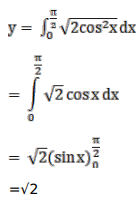# Mark against the correct answer in the following:Question:

Mark $(\sqrt{)}$ against the correct answer in the following:

$\int_{0}^{\pi / 2} \sqrt{1+\cos 2 x} d x=?$

A. $\sqrt{2}$

B. $\frac{3}{2}$

C. $\sqrt{3}$

D. 2

Solution: